SYLLABUS  Previous: 2.2.1 Interest rates: treasury  Up: 2.2 The credit market  Next: 2.2.3 Interest rate swaps

2.2.2 Underlying discount bonds and forward rates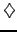[ SLIDE discount bond - spot rate - forward rate || same VIDEO as previous section: modem - LAN - DSL ]

The introductory section 1.3 suggested how fixed income securities, which pay a stream of coupons some time in the future ti>t   are a form of contingent claim that can always be replicated with a combination of zero-coupon bonds AP(t,ti).   Rather than the interest rate, it is the present value of such zero-coupon bonds that is traded on the bond market, with a spot price for each maturity date that is determined by the offer and demand from the investors. Given the similarity with the stock market, it is not surprising that most of the derivatives that have been discussed for shares can be generalized for bonds.

For simplicity, the principal is often normalized to unity A=1   and the discount bond P(t,T)   is used as a building block for more elaborate products. The discount function P(0,T)   in particular measures the present value of one unit due at a later time T; (2.2.2#fig.1) shows an example at a time when the treasury rate was relatively low and the market expects rising interest rates.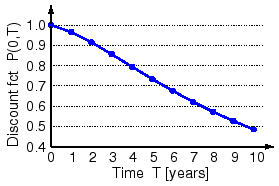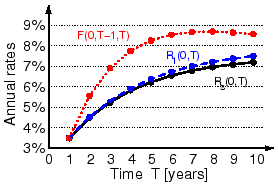For a short time, the spot rate r(t)   taken e.g. from the inter-bank market is nearly constant and the yield can be calculated without compounding Rs(t,T)   in (2.2.2#eq.1, left). For longer periods, a compounded calculation has to be used Rm(t,T)   in (2.2.2#eq.1, right) and is often replaced by a continuous compounding with a rate R(t,T)=exp[Y(t,T)]-1   calculated from the discount factor (2.2.2#eq.1, bottom)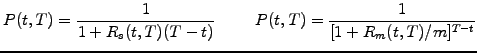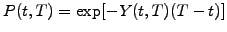(2.2.2#eq.1)

Plotted as a function of the time to maturity R(0,T),   these yield curves are often called the term structure of interest rates and can directly be constructed from the price of discount bonds quoted on the market (2.2.2#fig.1, 2.2.2#tab.1, exercise 2.09). Depending on whether the treasury rate is below or above the market expectations for the longer term interest rates, the term structure can have either a positive slope (as in fig.2.2.2#fig.1, right) or a negative slope.

From the ratio between values of the discount function in the future, it is convenient to define the implied forward rates, which correspond to the interest payed today (or any time t<T1<T2)   for a discount bond with a maturity T2   and starting in the future T1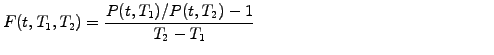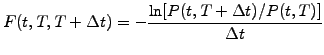(2.2.2#eq.2)

As expected, this definition recovers the present value for F(t,t,T)= R(t,T).   Examples of forward rates starting after a delay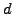are displayed in (2.2.2#fig.2) and have been derived from the same discount function that was used previously in (2.2.2#fig.1, 2.2.2#tab.1).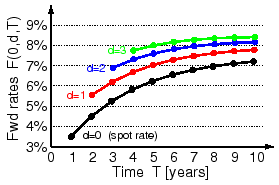Table 2.2.2#tab.1: Example of a discount function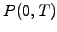and the corresponding present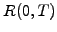and forward rates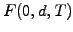starting after a delayfor a maturity date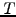.
 T [years]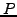(0,T)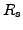(0,T)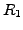(0,T)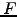(0,T-1,T)(0,1,T)(0,2,T)(0,3,T) 1 0.9662 0.0350 0.0350 0.0350 - - - 2 0.9153 0.0450 0.0452 0.0556 0.0556 - - 3 0.8563 0.0525 0.0531 0.0690 0.0620 0.0690 - 4 0.7947 0.0581 0.0591 0.0775 0.0668 0.0731 0.0775 5 0.7340 0.0623 0.0638 0.0826 0.0703 0.0760 0.0800 6 0.6762 0.0655 0.0674 0.0855 0.0729 0.0781 0.0817 7 0.6222 0.0679 0.0701 0.0867 0.0748 0.0796 0.0828 8 0.5725 0.0697 0.0722 0.0870 0.0761 0.0806 0.0835 9 0.5269 0.0710 0.0738 0.0865 0.0771 0.0812 0.0839 10 0.4853 0.0720 0.0750 0.0857 0.0778 0.0816 0.0841

Because of the uncertainty associated with the credit worthiness of long term borrowers and the seemingly random changes of the central bank policies, the price of a discount bond P(t,T), the yield Y(t,T)   and the forward rates F(t,T1,T2)   are all random functions of time via the spot rate r(t)   which will be discussed further in chapter 3. Nevertheless, is it possible for loan takers to protect themselves against unpredictable changes in the interest rate? Yes, using the so-called swaps and forward rate agreements.

SYLLABUS  Previous: 2.2.1 Interest rates: treasury  Up: 2.2 The credit market  Next: 2.2.3 Interest rate swaps